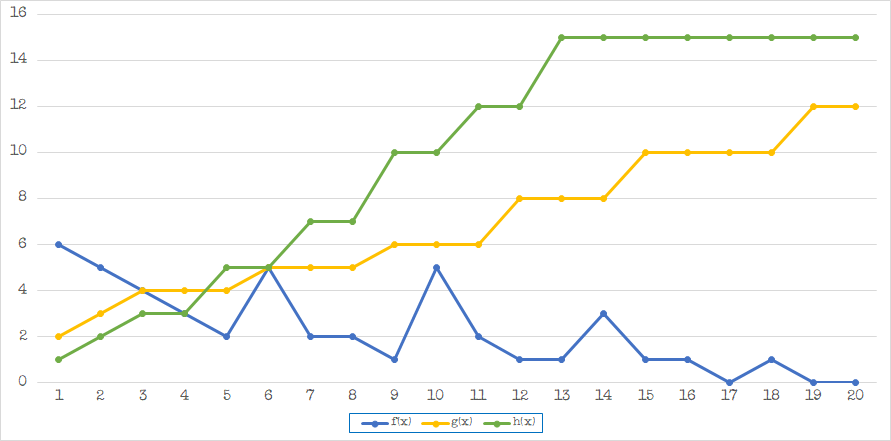## On the Subject of Forget Me Now

No, this is not a boss module. Stop being judgemental.

• This module has two screens, a main display on the bottom, and a stage counter on the right, and ten buttons numbered 0–9.
• To activate the module, press any of the numbered buttons.
• The number of stages the module has is equal to the number of modules on the bomb (not including needies). When the module has activated, it will display a number for all the stages one by one until all the stages have been shown.
• For each stage, add the displayed number and the added number received from Table 1, and take the least significant digit. This is the calculated number for that stage.
• After all the stages have been shown, press the calculated numbers on the numbered buttons in the order they were obtained.
• If an incorrect number is pressed for a stage, the module will strike and the displayed number for that stage will be shown with a light next to the corresponding numbered button. The stage display will also show the current stage number until the next correct input.
Let u[n] be the calculated numbers for each stage, let v[n] be the added numbers for each stage, and let n be the stage number. Let u[-1] be the last digit of the serial number, and u be the number on the button pressed to activate the module.

### Table 1:

 For each stage: If either u[n-2] or u[n-1] are 0, v[n] = ceil(h(n) * first digit of serial number / 5) Otherwise, if u[n-2] and u[n-1] are both even, v[n] = abs(g(n) * 4 - total stages) Otherwise, v[n] = u[n-2] + u[n-1] + digital root(f(n) * 2n²)

The function ceil() means to round the result up to the nearest integer. The function abs() means to take the absolute value of the result (remove negative signs). The function digital root() means to take the digital root of the result (the consective sum of the number’s digits until it reaches one digit).

### Table 2:

Use this graph to find the values of f(x), g(x), and h(x). Substitute n for x. Any value where x > 20 is treated as x = 20.